# Second DIMACS P-Hat Graph 3

The Second DIMACS Implementation Challenge: 1992-1993

Originator: Patrick Soriano and Michel Gendreau

NP Hard Problems: Maximum Clique, Graph Coloring, and Satisfiability, The Second DIMACS Implementation Challenge: 1992-1993.

Random graphs generated with the p hat generator which is a generalization of the classical uniform random graph generator. Uses 3 parameters: n, the number of nodes, and a and b, two density parameters verifying 0 <= a <= b <= 1.

## Examples

### Basic Examples

Retrieve the graph:

 In:=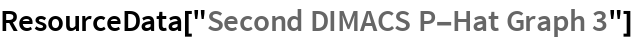Out=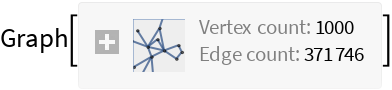Summary properties:

 In:=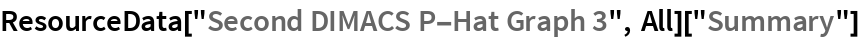Out=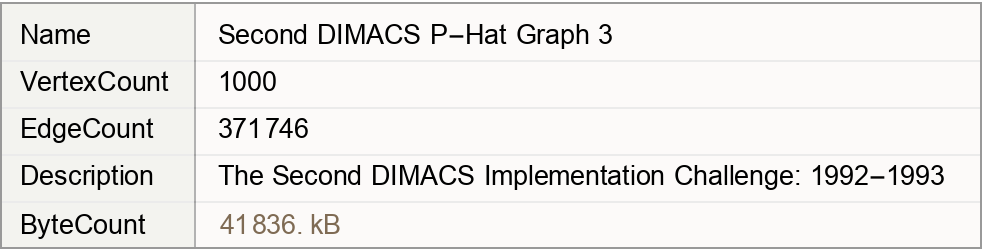### Basic Applications

Show the properties of the graph:

 In:=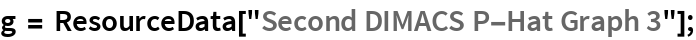In:=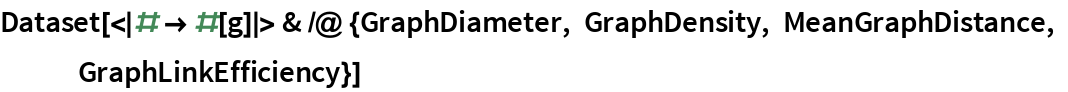Out=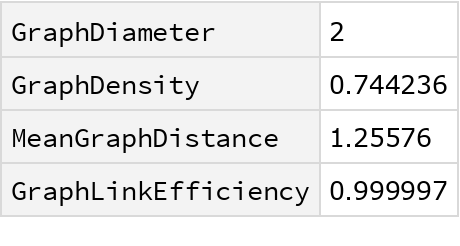Wolfram Research, "Second DIMACS P-Hat Graph 3" from the Wolfram Data Repository (2019)